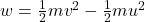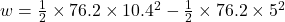Question

A water-skier is being pulled by a tow rope attached to a boat. As the driver pushes the throttle forward, the skier accelerates. A 76.2-kg water-skier has an initial speed of 5.0 m/s. Later, the speed increases to 10.4 m/s. Determine the work done by the net external force acting on the skier.

1.Work done will be equal to 3186.396 J

Explanation:

We have mass m = 76.2 kg

Initial velocity u = 5 m/sec

Final velocity v = 10.4 m/sec

We have to find the work done

From work energy theorem work done is equal to change in kinetic energyw = 3168.396 J

So work done will be equal to 3186.396 J